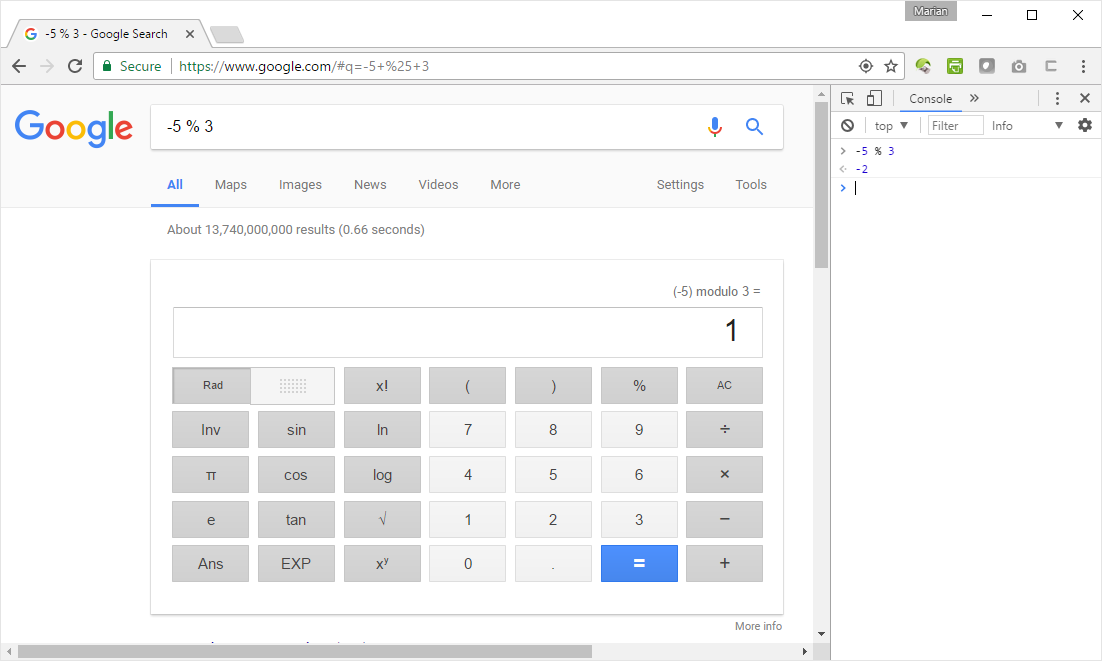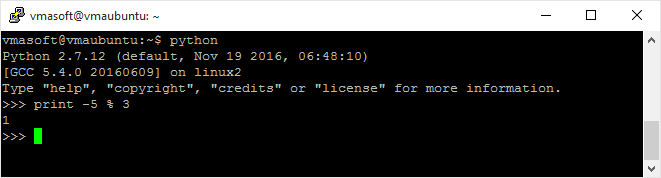# JavaScript Modulo operation and the Caesar Cipher

Successful implementation of a scientific calculation or algorithm is possible not only by understanding the features that a particular language or framework offers but also understanding the limitations.

Computers are precise scientific instruments but they do they work by manipulating entities in discrete spaces (you have a limited number of pixels on the screen, there is a limited numbers of bits that are behind each number, etc.)

Try to ignore the limitations or framework specs and soon you’ll find out that you have an impedance mismatch between your mathematical formula and the code you try to write.

## Modulo operator

Sometimes the situations is complicated by falsely advertised or understood framework functions or operators. This article focuses on the modulo operator.

Ask any C# or JavaScript programmer what is the modulo operator in their language and there is a big chance that they’ll gone answer: % (e.g. the percentage sign). Plenty of documentation refer to the % sign as modulo operator.

Wow! This is a subtle but very dangerous mistake. In C# and JavaScript % operator is used actually to calculate the remainder (with sign) left over when one operand is divided by the second operand. Therefore the operand should be correctly referred to as the signed remainder operator.

At first sight the signed remainder operator functions similarly to the modulo operator. Let’s do some tests by comparing the results returned by JavaScript with the ones returned by Google.

In Chrome, open the console (press F12 and select the Console tab). Type there, one by one, the calculations from the left column. Next type the same expressions in the Google search bar. Notice the results. They should be the same.

5 % 3 2 2
26 % 26 0 0
15 % 12 3 3

Let’s now try to use a negative value as the first operand:Surprise!

• -5 % 3 = 1 (according to Google)
• -5 % 3 = -2 (according to JavaScript)

Well … this shouldn’t be actually a surprise if we look at the definition of % operator in JavaScript (… or even C# or many other languages). Google calculates the true modulo, while these computer languages calculate a signed reminder.

However, not all programming languages / frameworks have the same implementation for %. In Python, for instance, the % operator calculates the true modulo in the same way as Google:This difference in behavior between languages may introduce subtle errors in your calculation, especially if you are trying to port an algorithm from one language to another!

## A problem understood is a problem half solved

Let’s suppose we need to implement a (scientific) calculation in JavaScript by using modulo arithmetic.

Since we now understand that JavaScript doesn’t have a true modulo operator, we can easily implement our modulo operation as a function.

There are multiple ways to implement modulo in JavaScript. I’ll show you 3 ways of doing it.

``````// Implement modulo by replacing the negative operand
// with an equivalent positive operand that has the same wrap-around effect
function mod(n, p)
{
if ( n < 0 )
n = p - Math.abs(n) % p;

return n % p;
}
``````
``````// Implement modulo by relying on the fact that the negative remainder
// is always p numbers away from a positive reminder
// Ex: -5 % 3 | -5 = -2 * 3 + 1 and -5 = -1 * 3 + (-2) | -2 + 3 = 1
function mod(n, p)
{
var r = n % p;

return r < 0 ? r + p : r;
}
``````
``````// Implement modulo by solving n = v * p + r equation
function mod(n, p)
{
return n - p * Math.floor( n / p );
}
``````

## Attack the problem

With more precise tools at our disposal, we are now ready to tackle that (scientific) calculation and expect to get correct results each and every time.

There are plenty of calculations that make use of modulo arithmetic… however for fun purposes I’ll show you how you can use modulo to implement Caesar Cipher – a very simple form of encryption, in which each letter in the original message is shifted to the left or right by a certain number of positions.

To decrypt the message we simply shift back the letters the same number of positions.

Example:

• JAVASCRIPT becomes MDYDVFULSW if we shift all letters by 3 positions
• MDYDVFULSW turns back to JAVASCRIPT if we shift back all letters by 3 positions.

If after shifting a letter goes outside the range of letters, then the letter is wrapped around in alphabet. Example: Letter Z becomes C if is shifted by 3 positions.

This “wrap-around” effect means use of modulo. In mathematical terms, the above can be expressed as this:

En(x) = (x + n) mod 26

Dn(x) = (x – n) mod 26

Trying to implement this algorithm in JavaScript without the use of a proper modulo operator will produce either incorrect results or a very cryptic and difficult to understand code.

By using any of mod functions defined above, the code expresses the mathematical equation identically:

``````// Function will implement Caesar Cipher to
// encrypt / decrypt the msg by shifting the letters
// of the message acording to the key
function encrypt(msg, key)
{
var encMsg = "";

for(var i = 0; i < msg.length; i++)
{
var code = msg.charCodeAt(i);

// Encrypt only letters in 'A' ... 'Z' interval
if (code >= 65 && code <= 65 + 26 - 1)
{
code -= 65;
code = mod(code + key, 26);
code += 65;
}

encMsg += String.fromCharCode(code);
}

return encMsg;
}
``````

Have fun! Encrypt a few messages to try out the code. Remember: If you encrypt with a positive key, use the complementary negative key to decrypt it.

You can also use this code to decode those ROT13 messages that appears everywhere on the web and newsgroups.

In a future article, I will tackle other ‘limitations’ and solutions to common languages and frameworks such as working correctly with floating point (IEEE 754) numbers in scientific calculations.

VMA

19 May 2017